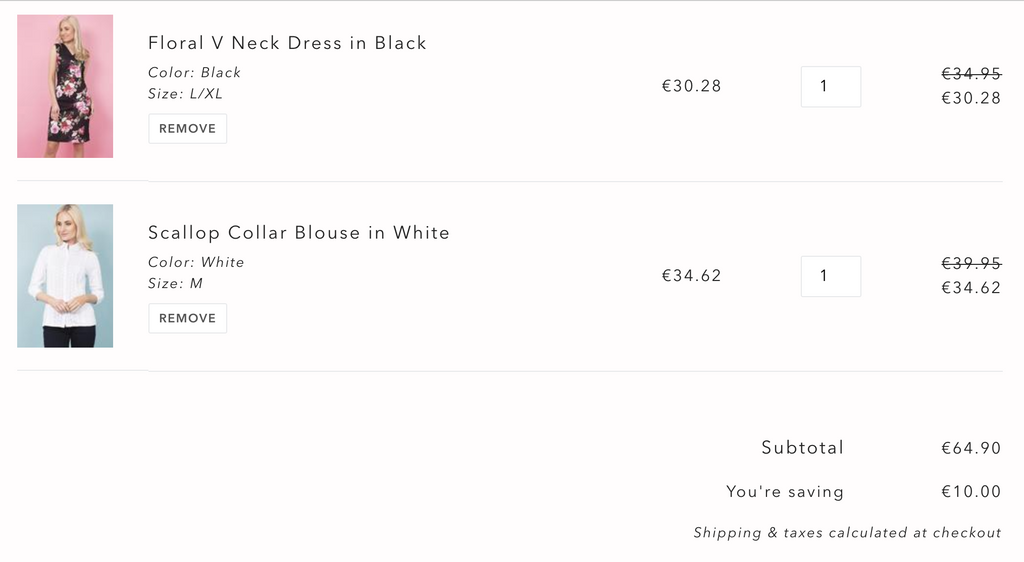# Shopify Scripts - Spend & Save Discount

Do you want to give a discount of \$Y for every \$X spent? This is known as a spend and save promotion and in this blog post we explain how you can implement this promotion with a Shopify script, which is only available to people using Shopify Plus. In this example, we want to give the customer a discount of \$10 for every \$50 that they spend and we only apply the discount to products that contain the "spend-and-save" tag. Here is what you can expect from implementing this script if you are using the Debut theme:If this looks good to you then continue reading to find out how it's implemented. To get started let's define the constants that we will be using for our Shopify script:

``````SPEND_THRESHOLD_AMOUNT = 5000
DISCOUNT_AT_THRESHOLD = 1000
ELIGIBLE_PRODUCT_TAG = "spend-and-save"
DISCOUNT_MESSAGE = ""
``````

Next, we need to iterate over our line items to determine which ones are eligible for the discount and to also calculate the total price of the eligible products which we will need in a second.

``````total_eligible_price = 0

# Loop over all the items to find eligble ones and total eligible discount price
eligible_items = Input.cart.line_items.select do |line_item|
total_eligible_price += Integer(line_item.line_price.cents.to_s)
product = line_item.variant.product
end
``````

Shopify scripts are very powerful and give you the ability to modify the pricing on a line item basis. This makes running a number of promotions much more viable to implement. However, in this case we want to apply a discount \$X on the entire cart, which is not directly available to us and requires a little workaround.

Here we indirectly apply the discount of \$X to the entire cart by distributing \$X across each eligible line item. On each iteration we carry forward the remainder which is pretty important as in a corner case where we have 3 products that cost €20 each this will result in a discount of \$3.33 on each of the three products which is a total of \$9.99 which is 1c the intended discount of \$10.

``````total_discount = (total_eligible_price/SPEND_THRESHOLD_AMOUNT).floor * DISCOUNT_AT_THRESHOLD

# Distribute the total discount across the products propotional to their price
remainder = 0.0
eligible_items.each do |line_item|
price = Integer(line_item.line_price.cents.to_s)
proportion =  price / total_eligible_price
discount_float = (total_discount * proportion) + remainder
discount = discount_float.round
remainder =  discount_float - discount
line_item.change_line_price(line_item.line_price - Money.new(cents: discount), message: DISCOUNT_MESSAGE) unless discount == 0
end

Output.cart = Input.cart
``````

Here is a link to the full script on Github.

Development
Shopify Plus
Shopify Scripts